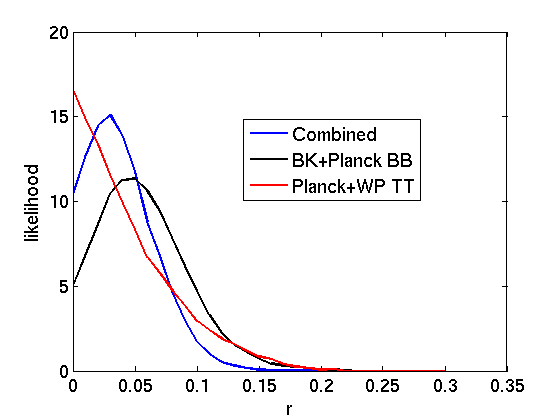## Tuesday, February 3, 2015

### Combined constraints from BICEP2, Keck, Planck and WMAP on primordial gravitational waves

This week, the joint analysis of BICEP2 (+ BICEP2's successor Keck) and Planck has finally arrived. The result is more or less what was expected, which is that what BICEP2 saw last year in the B-mode polarisation signal of the CMB was not actually primordial gravitational waves (as had originally been hoped and claimed), but was unfortunately actually due to dust in the Milky Way. Such is life. Though we did of course have the best part of a year to come to grips with this reality.Combined constraint on $$r$$ from polarisation and temperature measurements (in blue). Freshly digitised in the spirit of modern cosmology. Gives $$r\lesssim 0.09$$ at $$95\%$$ confidence.

As a result of subtracting the dust component in BICEP2/Keck's signal (obtained by comparing the measurements from BICEP2/Keck and Planck), the final constraint on the "tensor to scalar ratio" (or $$r$$) from the BICEP2/Keck measurement is that $$r<0.12$$ at $$95\%$$ confidence. This $$r$$ parameter essentially measures the amplitude of a primordial gravitational wave signal, so the net result is that the subtraction of dust takes BICEP2's high significance measurement of non-zero $$r$$ and converts it into simply an upper bound.

I've seen some comments on blogs, in media, on Twitter, etc that there is still evidence of some sort of excess signal in BICEP2/Keck over and above the dust, but I can't see any evidence of that in any of their published results. The final likelihood for $$r$$ (shown above in black) shows a plot consistent with $$r=0$$ at less than $$1-\sigma$$ (i.e. $$r=0$$ is less than one standard deviation away from the maximum likelihood value). In fact, it would seem that the measurement of the dust that has been obtained by comparing BICEP2/Keck's measurements with Planck's measurements has been so good that the B-mode constraint on $$r$$ from BICEP2/Keck is now competitive with (or even slightly better than) the constraint arising from temperature measurements of the CMB. This was always going to happen at some point in the future and it seems that this future has now arrived.